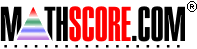Math Practice Online > free > lessons > Florida > 6th grade > Prime Factoring

## Prime Factoring

Drills you in factoring a number into its prime factors. For example, if you are to factor the number 60, your answer would be "2*2*3*5".

 Sample Problems for Prime Factoring Lesson for Prime Factoring

### This topic aligns to the following state standards

Grade 5: Num 2. Expresses a whole number as a product of its prime factors.
Grade 6: Num 3. Determines the prime factorization of a number less than or equal to 100.
Grade 7: Num 3. Determines the prime factorization of a composite number.

Copyright Accurate Learning Systems Corporation 2008.